## LCM and HCF

#### LCM and HCF

1. The LCM and the HCF of the numbers 28 and 42 are in the ratio :

1. L.C.M. of 28 and 42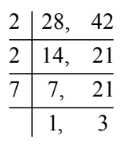L.C.M. of 28 and 42 = 2 × 2 × 7 × 3 = 84
H.C. F. of 28 and 42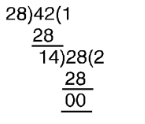∴ H.C. F = 14

##### Correct Option: A

L.C.M. of 28 and 42L.C.M. of 28 and 42 = 2 × 2 × 7 × 3 = 84
H.C. F. of 28 and 42∴ H.C. F = 14

 Required ratio = 84 = 6 : 1 14

1. Two pipes of length 1.5 m and 1.2 m are to be cut into equal pieces without leaving any extra length of pipes. The greatest length of the pipe pieces of same size which can be cut from these two lengths will be

1. Maximum length of each piece = HCF of 1.5 metre and 1.2 metre
First of all we find HCF of 1.5 metre and 1.2 metre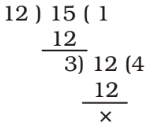∴ HCF of 1.5 and 1.2 metre = 0.3 metre

##### Correct Option: C

Maximum length of each piece = HCF of 1.5 metre and 1.2 metre
First of all we find HCF of 1.5 metre and 1.2 metre∴ HCF of 1.5 and 1.2 metre = 0.3 metre
Required Maximum length of each piece = 0.3 metre

1. In a school, 391 boys and 323 girls have been divided into the largest possible equal classes, so that each class of boys numbers the same as each class of girls. What is the number of classes ?

1. First of all we find HCF of 391 and 323.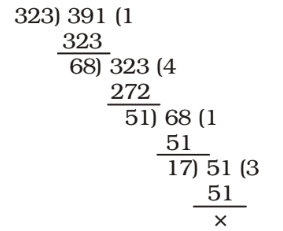##### Correct Option: D

First of all we find HCF of 391 and 323.∴ Number of classes = 17

1. Find the greatest number that will divide 390, 495 and 300 without leaving a remainder.

1. Required number = HCF of 390, 495 and 300
Firstly , we find HCF of 390, 495 and 300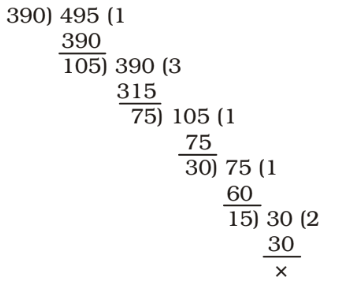##### Correct Option: B

Required number = HCF of 390, 495 and 300
Firstly , we find HCF of 390, 495 and 300HCF of 15 and 300 = 15
Hence , Required number = 15

1. The product of two 2–digit numbers is 2160 and their H.C.F. is 12. The numbers are

1. HCF of numbers = 12
Let the numbers be 12p and 12q where p and q are co–prime.
According to the question,
12p × 12q = 2160

 ⇒ pq = 2160 = 15 12 × 12

pq = 3 × 5 or 1 × 15
Here , p = 3 , q = 5

##### Correct Option: C

HCF of numbers = 12
Let the numbers be 12p and 12q where p and q are co–prime.
According to the question,
12p × 12q = 2160

 ⇒ pq = 2160 = 15 12 × 12

pq = 3 × 5 or 1 × 15
Here , p = 3 , q = 5
∴ Required numbers = 12 × 3 = 36 and 12 × 5 = 60